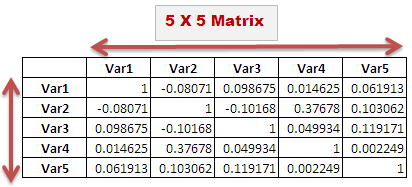# Excel Formula Explained : Correlation Matrix

This article explains how to compute a correlation matrix using an excel formula.

What is Correlation matrix?

It is simply defined as the measure of the relationship between two or more variables. Coefficients have a range of -1 to 1; -1 is the perfect negative correlation while +1 is the perfect positive correlation. It is also called multiple correlation coefficient. The post below explains how to calculate multiple correlation coefficient in Excel.

## Excel Formula to calculate Correlation Matrix

=CORREL(OFFSET(firstvariable_range,,ROWS(\$1:1)-1),OFFSET(firstvariable_range,,COLUMNS(\$A:A)-1))

For example, you have a 5 X 5 matrix and data for variables are entered in cells B3:F50 so paste this formula in cell J4
=CORREL(OFFSET(\$B\$3:\$B\$50,,ROWS(\$1:1)-1),OFFSET(\$B\$3:\$B\$50,,COLUMNS(\$A:A)-1))

In this case, firstvariable_range is B3:B50.

Download the workbook used in this example so you can copy and practice the formula.

How it works :
Let's take data for 5 variables and try to figure out the correlation between them.
1. CORREL Function
Usage : It returns the correlation coefficient between two variables.
Syntax : = CORREL ( first variable_range, second variable_range )
For variable 1 and variable 2, the syntax would be =CORREL(B3:B50, C3:C50)

2. OFFSET Function
Usage : It returns a reference to a range that is a given number of rows and columns from a given reference Syntax : = OFFSET (reference range, number of rows up or down, number of columns to the left or right)
For example, = OFFSET(\$B\$3:\$B\$50,,1) means one column to the right of B3:C50 i.e. C3:C50

3. ROWS / COLUMNS Function
Usage : It returns the number of rows or columns in a range. Syntax : = ROWS ( range ) For example, = ROWS (\$1:2) returns 2.OR = COLUMNS (\$A:B) returns 2
4. Dynamic CORREL Function
For variable 2 and variable 3,
=CORREL(OFFSET(\$B\$3:\$B\$50,,ROWS(\$1:2)-1),OFFSET(\$B\$3:\$B\$50,,COLUMNS(\$A:C)-1))
i. ROWS(\$1:2) returns 2. Hence, ROWS(\$1:2)-1 returns 1
ii. OFFSET(\$B\$3:\$B\$50,,ROWS(\$1:2)-1) returns reference of range C3:C50 i.e. data set for variable 2
iii. COLUMNS(\$A:C) returns 3. Hence, COLUMNS(\$A:C)-1 returns 2
iv. OFFSET(\$B\$3:\$B\$50,,COLUMNS(\$A:C)-1) returns reference of variable 3How to use it
Paste the formula below to N rows x N columns. In 5 X 5 matrix , paste down to 5 rows and right to 5 columns.
`=CORREL(OFFSET(\$B\$3:\$B\$50,,ROWS(\$1:1)-1),OFFSET(\$B\$3:\$B\$50,,COLUMNS(\$A:A)-1))`
Related Posts
ShareDeepanshu founded ListenData with a simple objective - Make analytics easy to understand and follow. He has over 10 years of experience in data science. During his tenure, he worked with global clients in various domains like Banking, Insurance, Private Equity, Telecom and HR.

6 Responses to "Excel Formula Explained : Correlation Matrix"
1.Awesome, this saved me tons of time! Thank you for the step-by-step instructions.

2.Thanks a lot!!

3.THANK YOU VERY MUCH HELPED A LOT

4.5.6.